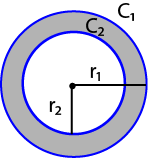## Annulus Calculator

 r1 = r2 = Units: C1 = 0 C2 = 0 A1 = 0 A2 = 0 A0 = 0C1 = outer circumference
C2 = inner circumference
A1 = area of circle of r1
A2 = area of circle of r2

The online annuluses calculator to find the area, circumference and radius of an annulus. When you know two known variable, then select from the droplist, calculate the other 5 unknowns.

### Annulus Formulas:

Given r1 and r2:
C1 = 2πr1
C2 = 2πr2
A1 = πr12
A2 = πr22
A0 = A1 - A2.

Given r1 and C2:
r2 = C2 / 2π
C1 = 2πr1
A1 = πr12
A2 = πr22
A0 = A1 - A2.

Given r1 and A2:
r2 = √(A2 / π)
C1 = 2πr1
C1 = 2πr2
A1 = πr12
A0 = A1 - A2.

Given r2 and C1:
r1 = C1 / 2π
C2 = 2πr2
A1 = πr12
A2 = πr22
A0 = A1 - A2.

Given C1 and C2:
r1 = C1 / 2π
r2 = C1 / 2π
A1 = πr12
A2 = πr22
A0 = A1 - A2.

Given C1 and A2:
r1 = C1 / 2π
r2 = √(A2 / π)
C2 = 2πr2
A1 = πr12
A0 = A1 - A2.

Given r2 and A1:
r1 = √(A1 / π)
C1 = 2πr1
C2 = 2πr2
A2 = πr22
A0 = A1 - A2.

Given C2 and A1:
r1 = √(A1 / π)
r2 = C2 / 2π
C1 = 2πr1
A2 = πr22
A0 = A1 - A2.

Given A1 and A2:
r1 = √(A1 / π)
r2 = √(A2 / π)
C1 = 2πr1
C2 = 2πr2
A0 = A1 - A2.

Given r1 and A0:
C1 = 2πr1
A1 = πr12
A2 = A1 - A0
r2 = √(A2 / π)
C2 = 2πr2.

Given r2 and A0:
C2 = 2πr2
A2 = πr22
A1 = A0 + A2
r1 = √(A1 / π)
C1 = 2πr2.

Given C1 and A0:
r1 = C1 / 2π
A1 = πr12
A2 = A1 - A0
r2 = √(A2 / π)
C2 = 2πr2.

Given C2 and A0:
r2 = C2 / 2π
A2 = πr22
A1 = A0 + A2
r1 = √(A1 / π)
C1 = 2πr1.

Given A1 and A0:
A2 = A1 - A0
r1 = √(A1 / π)
r2 = √(A2 / π)
C1 = 2πr1
C2 = 2πr2.

Given A2 and A0:
A1 = A0 + A2
r1 = √(A1 / π)
r2 = √(A2 / π)
C1 = 2πr1
C2 = 2πr2.

Thinkcalculator.com provides you helpful and handy calculator resources.